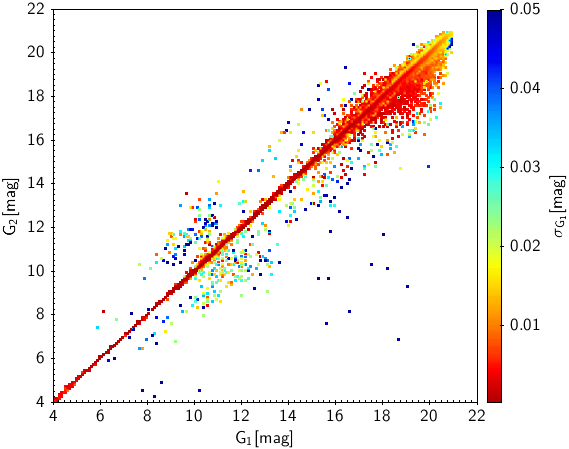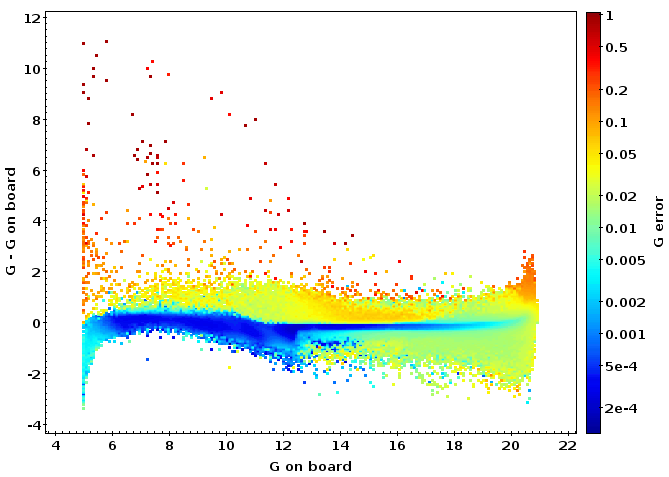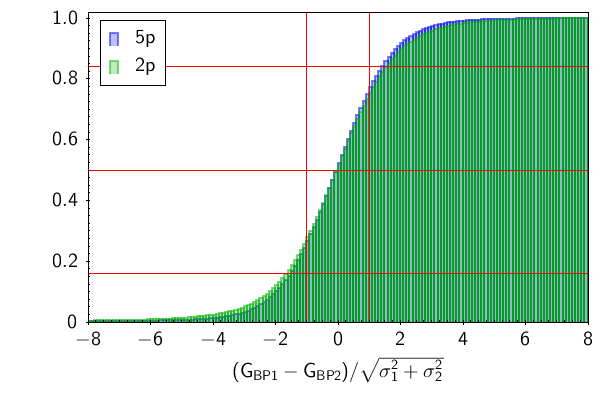# 10.2.5 Photometry

The validation of the $G$, $G_{\rm BP}$ and $G_{\rm RP}$ photometry is already described in much detail in Evans et al. (2018) and Arenou et al. (2018). An important point is that the $G_{\rm BP}$ and $G_{\rm RP}$ photometry in Gaia DR2 is a simple diaphragm photometry of prism spectra, assuming the source to be an isolated point source. Blending issues therefore arise for binaries as well as for sources in crowded regions. The specific issues for the observed colours due to blending are also discussed in Section 5.5.2. Blending is of much less concern for the $G$ photometry, which is derived from image fitting.

A common issue for all three bands is their sensitivity to any imperfection in the estimation of the sky background and the electronic bias. Again, $G_{\rm BP}$ and $G_{\rm RP}$ are the more affected bands because of their five times larger data acquisition windows and because of their even weight to all samples.Figure 10.10: G magnitudes for duplicated sources. Left: both have full astrometric solution; right: at least one of them has only two parameter astrometry. The abscissa gives the solution that was removed and the ordinate the one that was kept. The colour coding shows the estimated uncertainty on the magnitude of the source that was removed.Figure 10.11: Deviation of the (weighted) G magnitude from the mean of the (unweighted) onboard detections. The colour coding indicates the uncertainty on G as derived from the flux uncertainty.

We have already mentioned in Section 10.2.2 that some sources had two independent solutions right until the last stages of the Gaia DR2 processing. Figure 10.10 shows the two $G$ magnitude values for these duplicates. When we have two, full astrometric solutions (left panel) the agreement between the two $G$ magnitudes is very good and only a very small fraction fall outside the diagonal. Also for the cases involving two parameter solutions in astrometry, the vast majority of sources fall in fact on the diagonal, and even the solutions to be removed have small, estimated errors. However, the fraction of problematic solutions is higher is this case. At 2–3 mag from the diagonal lie bands of large amplitude variables, where a too large weight on the magnitude in the cross match procedure used for Gaia DR2 has led to a split into a brighter and a fainter solution. The lightcurves published in Gaia DR2 will then only contain either the brighter or the fainter part. More puzzling is the group of cases where bright sources, e.g. around 8 mag, are sometimes seen as very faint, around 16 mag. These are mostly processing errors involving insufficient outlier rejection and a too high weight to a faint, spurious detection near the bright source, and it is worth remembering that such cases are rare. When occurring in a duplicated source, the solution with the poorer astrometry is removed. As the figure shows, this solution is also the one with the spurious photometry. This type of error must, however, also be expected among the general source population. A notorious example is the star RR Lyr which comes out almost 10 magnitudes too faint. This issue was discovered too late to be corrected in Gaia DR2. As can be seen Figure 10.11 there are very few of these extreme cases and they all have large estimated uncertainties.

The solutions for the duplicated sources offer an obvious opportunity for an internal test of the estimated flux errors. Figure 10.12, Figure 10.13 and Figure 10.14 show the accumulated, normalised differences for the three bands. Note that this comparison was made in magnitudes instead of in flux. Especially for the $G$ magnitude the distribution deviates strongly from the expected Gaussian. However, if we include an error floor of a few mmag, we get near Gaussian distributions for the well-behaved sources with full, five-parameter, astrometric solutions. The reason for this error floor is not fully understood, but it suggests that the photometric error model needs improvement.

Sources demoted to only two astrometric parameters are either very faint or have some intrinsic problem (e.g., binarity) and can therefore not be expected to have the same, benign properties as the majority of sources. The difference is striking for the $G$ band, whereas it is much more modest for the $G_{\rm BP}$ and $G_{\rm RP}$ bands. Two effects contribute here. For a close binary, the observed flux in the $G$ band depends heavily on the scan direction, whereas the acquisition windows for $G_{\rm BP}$ and $G_{\rm RP}$ will include almost all the signal independently of the scan direction and the mean fluxes will tend to be better defined. Another factor is that the $G_{\rm BP}$ and $G_{\rm RP}$ bands generally have larger estimated errors than the $G$ band and therefore are less sensitive to small imperfections in the instrument calibration.Figure 10.12: Normalised, accumulated G magnitude differences for duplicated sources brighter than 17 mag. The horizontal lines indicate the levels of the mean values plus and minus one standard deviation for a Gaussian distribution. Left: direct normalisation with the magnitude uncertainties. Right: normalisation including an error floor of 2.3 mmag.Figure 10.13: Normalised, accumulated GBP magnitude differences for duplicated sources brighter than G = 17 mag. Left: direct normalisation with the magnitude errors. Right: normalisation including an error floor of 2.3 mmag.Figure 10.14: Normalised, accumulated GRP magnitude differences for duplicated sources brighter than G = 17 mag. Left: direct normalisation with the magnitude errors. Right: normalisation including an error floor of 2.3 mmag.